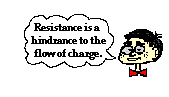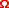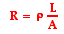Current Electricity - Lesson 3 - Electrical Resistance

# Resistance

An electron traveling through the wires and loads of the external circuit encounters resistance. Resistance is the hindrance to the flow of charge. For an electron,the journey from terminal to terminal is not a direct route. Rather, it is a zigzag path that results from countless collisions with fixed atoms within the conducting material. The electrons encounter resistance - a hindrance to their movement. While the electric potential difference established between the two terminals encourages the movement of charge, it is resistance that discourages it. The rate at which charge flows from terminal to terminal is the result of the combined effect of these two quantities.

### Variables Affecting Electrical Resistance

The flow of charge through wires is often compared to the flow of water through pipes. The resistance to the flow of charge in an electric circuit is analogous to the frictional effects between water and the pipe surfaces as well as the resistance offered by obstacles that are present in its path. It is this resistance that hinders the water flow and reduces both its flow rate and its drift speed. Like the resistance to water flow, the total amount of resistance to charge flow within a wire of an electric circuit is affected by some clearly identifiable variables.

First, the total length of the wires will affect the amount of resistance. The longer the wire, the more resistance that there will be. There is a direct relationship between the amount of resistance encountered by charge and the length of wire it must traverse. After all, if resistance occurs as the result of collisions between charge carriers and the atoms of the wire, then there is likely to be more collisions in a longer wire. More collisions mean more resistance.

Second, the cross-sectional area of the wires will affect the amount of resistance. Wider wires have a greater cross-sectional area. Water will flow through a wider pipe at a higher rate than it will flow through a narrow pipe. This can be attributed to the lower amount of resistance that is present in the wider pipe. In the same manner, the wider the wire, the less resistance that there will be to the flow of electric charge. When all other variables are the same, charge will flow at higher rates through wider wires with greater cross-sectional areas than through thinner wires.

A third variable that is known to affect the resistance to charge flow is the material that a wire is made of. Not all materials are created equal in terms of their conductive ability. Some materials are better conductors than others and offer less resistance to the flow of charge. Silver is one of the best conductors but is never used in wires of household circuits due to its cost. Copper and aluminum are among the least expensive materials with suitable conducting ability to permit their use in wires of household circuits. The conducting ability of a material is often indicated by its resistivity. The resistivity of a material is dependent upon the material's electronic structure and its temperature. For most (but not all) materials, resistivity increases with increasing temperature. The table below lists resistivity values for various materials at temperatures of 20 degrees Celsius.

 Material Resistivity (ohm•meter) Silver 1.59 x 10-8 Copper 1.7 x 10-8 Gold 2.2 x 10-8 Aluminum 2.8 x 10-8 Tungsten 5.6 x 10-8 Iron 10 x 10-8 Platinum 11 x 10-8 Lead 22 x 10-8 Nichrome 150 x 10-8 Carbon 3.5 x 10-5 Polystyrene 107 - 1011 Polyethylene 108 - 109 Glass 1010 - 1014 Hard Rubber 1013

As seen in the table, there is a broad range of resistivity values for various materials. Those materials with lower resistivities offer less resistance to the flow of charge; they are better conductors. The materials shown in the last four rows of the above table have such high resistivity that they would not even be considered to be conductors.

### Look it Up!

Use the Resistivity of a Material widget to look up the resistivity of a given material. Type the name of the material and click the Submit button to find its resistivity.

### Mathematical Nature of Resistance

Resistance is a numerical quantity that can be measured and expressed mathematically. The standard metric unit for resistance is the ohm, represented by the Greek letter omega -. An electrical device having a resistance of 5 ohms would be represented as R = 5. The equation representing the dependency of the resistance (R) of a cylindrically shaped conductor (e.g., a wire) upon the variables that affect it iswhere L represents the length of the wire (in meters), A represents the cross-sectional area of the wire (in meters2), andrepresents the resistivity of the material (in ohm•meter). Consistent with the discussion above, this equation shows that the resistance of a wire is directly proportional to the length of the wire and inversely proportional to the cross-sectional area of the wire. As shown by the equation, knowing the length, cross-sectional area and the material that a wire is made of (and thus, its resistivity) allows one to determine the resistance of the wire.

### Investigate!

Resistors are one of the more common components in electrical circuits. Most resistors have stripes or bands of colors painted on them. The colors reveal information about the resistance value. Perhaps you're doing a lab and need to know the resistance of a resistor used in the lab. Use the widget below to determine the resistance value from the colored stripes.

### Check Your Understanding

1. Household circuits are often wired with two different widths of wires: 12-gauge and 14-gauge. The 12-gauge wire has a diameter of 1/12 inch while the 14-gauge wire has a diameter of 1/14 inch. Thus, 12-gauge wire has a wider cross section than 14-gauge wire. A 20-Amp circuit used for wall receptacles should be wired using 12-gauge wire and a 15-Amp circuit used for lighting and fan circuits should be wired using 14-gauge wire. Explain the physics behind such an electrical code.

2. Based on the information stated in the above question, explain the risk involved in using 14-gauge wire in a circuit that will be used to power an 16-ampere power saw.

3. Determine the resistance of a 1-mile length of 12-gauge copper wire. Given: 1 mile = 1609 meters and diameter = 0.2117 cm.

4. Two wires - A and B - with circular cross-sections have identical lengths and are made of the same material. Yet, wire A has four times the resistance of wire B. How many times greater is the diameter of wire B than wire A?

Next Section: